JEE  >  Class 12 Chemistry Sample paper Test - 1 (with solution)

# Class 12 Chemistry Sample paper Test - 1 (with solution) | Additional Study Material for JEE

 1 Crore+ students have signed up on EduRev. Have you?

Ques 1.  Among octahedral and tetrahedral crystal fields in which case the magnitude of crystal field splitting is larger?
Ans. Octahedral crystal field.
Ques 2. Why do amines act as nucleophiles?
Ans. Due to the presence of lone pair of electron in nitrogen atom
Ques 3. What are monosaccharides?
Ans. A carbohydrate that cannot be further hydrolysed to give simplar unit of polyhydroxy aldehyde on ketone is called monosaccharide, e.g.. Glucose, ribose etc
Ques 4. Why is para nitrophenol is more acidic than phenol?
Ans. Due to presence of  -NO2 group, it increases the positive charge density on oxygen atom.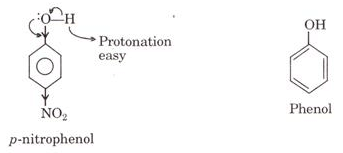Ques 5. Why is FeClpreffered over KCl in case of a cut leading to bleeding?
Ans.  Fe3+ is better coagulating ion due to greater positive charge on it.
Ques 6.
Write the IUPAC name of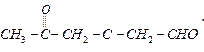Ans.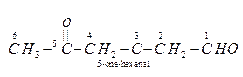Ques 7. Write the balanced equation for complete hydrolysis of XeF6.
Ans.  XeF6+3H20 → XeO2+6HF
Ques 8. How is half-life period related to initial concentration for a second order reaction?
Ans.  The half-life of a 2nd order reaction is inversely proportional to the initial concentration of the reactants.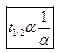Ques 9. Classify each of the following as being either a p-type or an n-type semiconductor. Give reason. (a) Si doped with In.  (b) si doped with P.
Ans.    (a) p-type because it has 3-valence electrons. Holes are produced which can move through the crystal like a (+)ve charge.
(b) n-type, because p-type has 5 valence electrons. The 5th electron becomes delocalised and is free to contribute to electrical conduction.
Ques 10.  Consider the decomposition of  H2Oin alkaline medium which is catalyzed by iodide ions.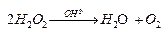This reaction takes place in two steps:
1st step:  H2O+ I- → H2O + IO-(Slow) H2O2 + I- + O2(Fast)
(a) What is the molecularity of each individual step?
(b) Write the rate law expression and determine the order of reaction w.r.t. H2O2

Ans.
Ques 11.  Complete the following reactions :

(a) (NH4)Cr2O2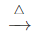(b) I-+O2+H2O →
Ans.
(a)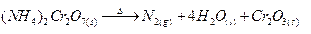(b)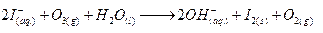Ques 12. An aromatic compound (A) on treatment with ammonia followed by heating forms compound (B) which on heating with Br2 and KOH forms a compound (C) having molecular formula ( C6H6N). Give the structure of A, B and C. Write the equation involved.
Ans.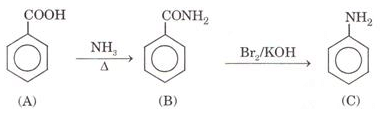Ques 13. Draw the structure of the following :
(a) HOClO2
(b) XeO
2
Ans.

(a)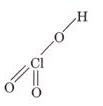(b)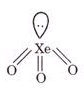Ques 14.  Using Valance bond theory. Predict the geometry and magnetic character of  [NiCl4]2-
Ans.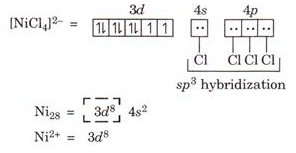Geometry: Tetrahedral due to sp3  hybridization
Magnetic character:  Paramagnetic due to presence of two unpaired electron.
Ques 15.
Give reasons :
(a) Rusting of iron pipe can be prevented by joining it with a piece of magnesium.

(b) Conductivity of an electrolyte solution decreases with the decrease in concentration.
Ans.
(a) Rusting of iron is prevented in the given case due to cathodic protection in which magnesium metal is oxidised in presence to iron and acts as anode.
(b) When the concentration decreases the number of ions present in unit volume of solution decreases as a result electrical conductivity decreases.
Ques 16. Identify A and B in. the following:
(i)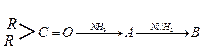(ii)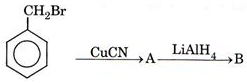Ans.

Ques 17. Write the Nernst equation and calculate the e.m.f. of the following cell at 298K :
Ans.

Ques 18. (a) What is meant by 'Point defects' in crystal?
(b) Define dislocation in crystal.

Ans. (a) Point defects: Defect caused by missing or misplaced ions or atoms in the crystal lattice are known as point defects in the crystal.
(b) When a crystal point is missing from its normal lattice position but occupies position in interstitials is known as dislocation in crystal.

Ques 19. Give one example of each of the following :
(a) An artificial sweetner.
(b) A non-ionic detergent.
(c) A pain reliever used for relief from post-operative pain.

Ans:  (a) Asparlaine is an artificial sweetner.
(b) Non-ionic detergent : Ester of stearic acid and polyethylene glycol.
(c) Morphine acts as pain reliever.

The document Class 12 Chemistry Sample paper Test - 1 (with solution) | Additional Study Material for JEE is a part of the JEE Course Additional Study Material for JEE.
All you need of JEE at this link: JEE

## Additional Study Material for JEE

22 videos|162 docs|17 tests

## Additional Study Material for JEE

22 videos|162 docs|17 tests

Track your progress, build streaks, highlight & save important lessons and more!(Scan QR code)

,

,

,

,

,

,

,

,

,

,

,

,

,

,

,

,

,

,

,

,

,

;